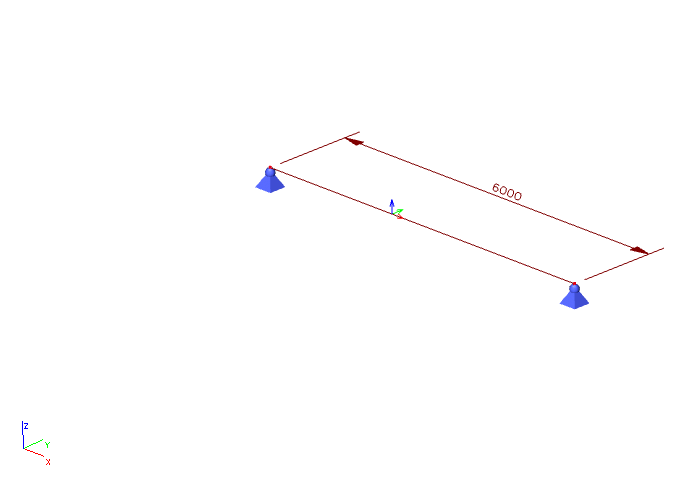# ResultInternalForce1D¶

Internal force 1D

Internal forces on line, beam, member. Result in member axis (not in principal axis).## Specification in the excel¶

Data type

Example / enum definition

Required

Description

Result on

Enum

On beam

On rib

yes

Specify object where the result is

On beam - StructuralCurveMember

On rib - StructuralCurveMemberRib

Member

String

B1

yes, if Result on = On beam

Reference to the name of 1D member - StructuralCurveMember

Member Rib

String

B2

yes, if Result on = On rib

Reference to the name of 1D member - rib StructuralCurveMemberRib

Result for

Enum

yes

Specifies from where the result is coming from (from Load Case, Load Combination)

String

LC1

yes, if Result for = Load case

Reference to the name of StructuraLoadCase

String

COM1

yes, if Result for = Combination

Reference to the name of StructuralLoadCombination

Combination key

String

1.35*LC1+1.5*LC2+1.5*LC3+1.5*LC4

no

Allows to define exact combination per result section

Structure of string:

For envelopes and national standard (code) combinations, this column specifies for which exact combination is the result

Section at [m]

Double

0.100

yes

X coordinate on the beam (distance from the start node) where the result is located

Index

Integer

1

yes

Index of the section on beam. See notes.

N [kN]

Double

3.00

yes

Result value of N

(Normal force)

Vy [kN]

Double

3.00

yes

Result value of Vy

(Shear force in Y axis direction)

Vz [kN]

Double

3.00

yes

Result value of Vz

(Shear force in Z axis direction)

Mx [kNm]

Double

0.000

yes

Result value of Mx

(Moment around X axis)

My [kNm]

Double

4.500

yes

Result value of My

(Moment around Y axis)

Mz [kNm]

Double

4.500

yes

Result value of Mz (Moment around Z axis)